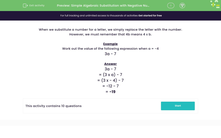# Use Simple Algebraic Substitution with Negative Numbers

In this worksheet, students will substitute negative numbers for letters and work out the value of simple expressions with one variable.Key stage:  KS 3

Curriculum topic:   Algebra

Curriculum subtopic:   Substitute Numerical Values for Formulae/Expressions

Popular topics:   Algebra worksheets, Year 7 Algebra worksheets

Difficulty level:#### Worksheet Overview

When we substitute a number for a letter, we simply replace the letter with the number.

However, we must remember that 4b means 4 x b.

Example

Work out the value of the following expression when a = 4

3a - 7

3a - 7

3a just means 3 x a , so we have

3 x a - 7

Change the letter (a) into the number we've been given (4)

3 x 4 - 7

Now multiply first - remember the order of BIDMAS (or BODMAS)

12 - 7 = 5

Sometimes we have a negative number to substitute and then we need to be very careful about the correct signs!

Example

Work out the value of the following expression when a = -4

3a - 7

3a - 7

3a just means 3 x a , so we have

3 x a - 7

Change the letter (a) into the number we've been given (-4)

3 x -4 - 7

Now multiply first - remember the order of BIDMAS (or BODMAS)

3 x -4 = -12

Finally, subtract 7:

-12 - 7 = -19

When working with negatives, it's important to remember the rules!

Two negative signs next to each other means a positive.

One negative and one positive next to each other means a negative.

If we multiply or divide one positive and one negative, we get a negative.

If we multiply two positives or two negatives, we get a positive.

Are you happy with this?### What is EdPlace?

We're your National Curriculum aligned online education content provider helping each child succeed in English, maths and science from year 1 to GCSE. With an EdPlace account you’ll be able to track and measure progress, helping each child achieve their best. We build confidence and attainment by personalising each child’s learning at a level that suits them.

Get started